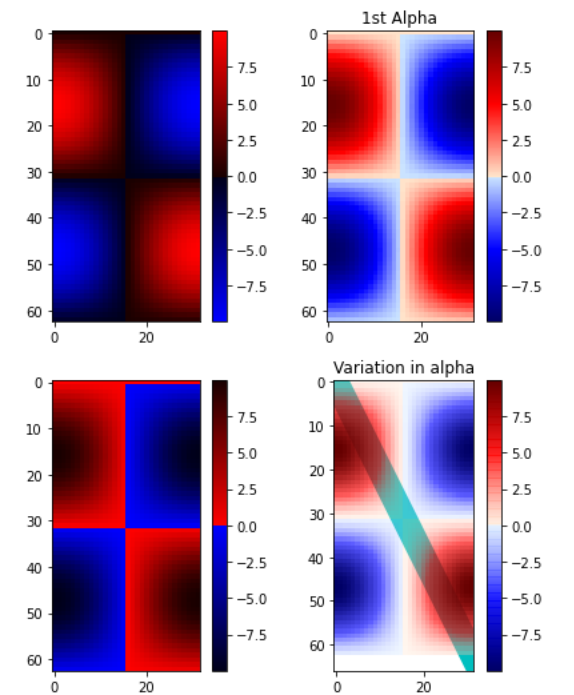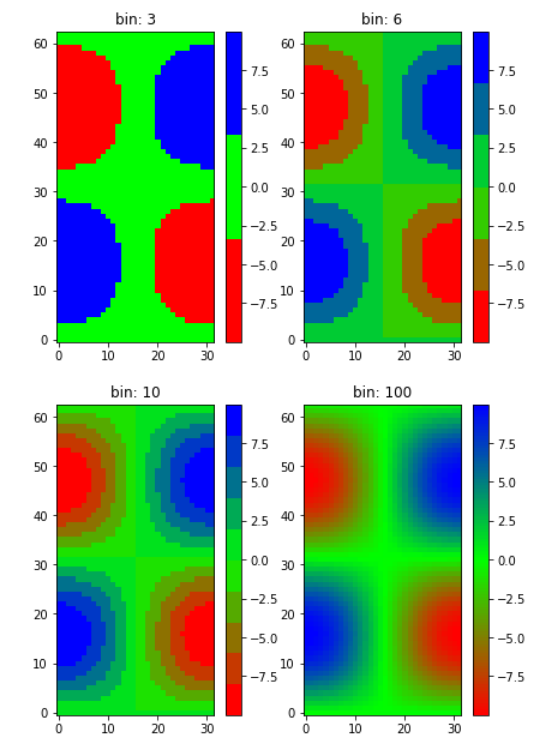# Matplotlib.colors.LinearSegmentedColormap class in Python

Matplotlib is an amazing visualization library in Python for 2D plots of arrays. Matplotlib is a multi-platform data visualization library built on NumPy arrays and designed to work with the broader SciPy stack.

## matplotlib.colors.LinearSegmentedColarmap

The matplotlib.colors.LinearSegmentedColarmap class belongs to the matplotlib.colors module. The matplotlib.colors module is used for converting color or numbers arguments to RGBA or RGB.This module is used for mapping numbers to colors or color specification conversion in a 1-D array of colors also known as colormap.

The matplotlib.colors.LinearSegmentedColormap class is used to colormap objects based on lookup tables with the help of linear segments. The table for the lookup is generated by linear interpolation for every primary color as the 0-1 domain divide it into any number of segments. It can also be used for creating a color map from linear mapping segments. A dictionary by the name of segment data is present with a red, blue and green entries. Every entry needs to be a list of x, y0, y1 tuples, creating rows of the table. It is important to note that alpha entries are optional.
For instance assume you want red to increment from 0 to 1, green to do the same but over the middle half and the blue over the top half. Then you would use the following dictionary:

seg_data_dict =

{‘red’: [(0.0, 0.0, 0.0),
(0.5, 1.0, 1.0),
(1.0, 1.0, 1.0)],

‘green’: [(0.0, 0.0, 0.0),
(0.25, 0.0, 0.0),
(0.75, 1.0, 1.0),
(1.0, 1.0, 1.0)],

‘blue’: [(0.0, 0.0, 0.0),
(0.5, 0.0, 0.0),
(1.0, 1.0, 1.0)]}

Every row in the table for a given color is a sequence of the tuple x, y0, y1. In every sequence, the x must increment monotonically from 0to 1. For all input value z falling between x[i] and x[i+1], the linearly interpolated output value of a given color is between y1[i] and y0[i+1]:

row i: x y0 y1
row i+1: x y0 y1

Therefore, y0 in the first row and y1 in the last row is never used.

Methods of the class:

1. static from_list(name, colors, N=256, gamma=1.0): This method is used to create a linear segmented colormap with name from a sequence of colors which evenly move from colors at val=0 to colors[-1] at val=1. N represents the number of rgb quantization levels.Also, list of tuples(value, color) can create division in the range unevenly.
2. reversed(self, name=None): It is used to make reversed instances of the Colormap.
parameters:

• name: It is an optional parameter taht accepts the name for the reversed colormap in the form of a string. If None, the name sets to the name of the parent colormap + “r”.

Returns: This method returns a reversed colormap.

3. set_gamma(self, gamma): It is used to regenerate a color map by setting a new gamma value

Example 1:

 `import` `numpy as np ` `import` `matplotlib.pyplot as plt ` `from` `matplotlib.colors ``import` `LinearSegmentedColormap ` ` `  `# some dummy data ` `a ``=` `np.arange(``0``, np.pi, ``0.1``) ` `b ``=` `np.arange(``0``, ``2` `*` `np.pi, ``0.1``) ` `A, B ``=` `np.meshgrid(a, b) ` `X ``=` `np.cos(A) ``*` `np.sin(B) ``*` `10` ` `  `# custom segmented color dictionary ` ` `  `cdict1 ``=` `{``'red'``:   ((``0.0``, ``0.0``, ``0.0``), ` `                   ``(``0.5``, ``0.0``, ``0.1``), ` `                   ``(``1.0``, ``1.0``, ``1.0``)), ` ` `  `         ``'green'``: ((``0.0``, ``0.0``, ``0.0``), ` `                   ``(``1.0``, ``0.0``, ``0.0``)), ` ` `  `         ``'blue'``:  ((``0.0``, ``0.0``, ``1.0``), ` `                   ``(``0.5``, ``0.1``, ``0.0``), ` `                   ``(``1.0``, ``0.0``, ``0.0``)) ` `        ``} ` ` `  `cdict2 ``=` `{``'red'``:   ((``0.0``, ``0.0``, ``0.0``), ` `                   ``(``0.5``, ``0.0``, ``1.0``), ` `                   ``(``1.0``, ``0.1``, ``1.0``)), ` ` `  `         ``'green'``: ((``0.0``, ``0.0``, ``0.0``), ` `                   ``(``1.0``, ``0.0``, ``0.0``)), ` ` `  `         ``'blue'``:  ((``0.0``, ``0.0``, ``0.1``), ` `                   ``(``0.5``, ``1.0``, ``0.0``), ` `                   ``(``1.0``, ``0.0``, ``0.0``)) ` `        ``} ` ` `  `cdict3 ``=` `{``'red'``:  ((``0.0``, ``0.0``, ``0.0``), ` `                   ``(``0.25``, ``0.0``, ``0.0``), ` `                   ``(``0.5``, ``0.8``, ``1.0``), ` `                   ``(``0.75``, ``1.0``, ``1.0``), ` `                   ``(``1.0``, ``0.4``, ``1.0``)), ` ` `  `         ``'green'``: ((``0.0``, ``0.0``, ``0.0``), ` `                   ``(``0.25``, ``0.0``, ``0.0``), ` `                   ``(``0.5``, ``0.9``, ``0.9``), ` `                   ``(``0.75``, ``0.0``, ``0.0``), ` `                   ``(``1.0``, ``0.0``, ``0.0``)), ` ` `  `         ``'blue'``:  ((``0.0``, ``0.0``, ``0.4``), ` `                   ``(``0.25``, ``1.0``, ``1.0``), ` `                   ``(``0.5``, ``1.0``, ``0.8``), ` `                   ``(``0.75``, ``0.0``, ``0.0``), ` `                   ``(``1.0``, ``0.0``, ``0.0``)) ` `        ``} ` ` `  `# Creating a modified version of cdict3  ` `# with some transparency ` `# in the center of the range. ` `cdict4 ``=` `{``*``*``cdict3, ` `          ``'alpha'``: ((``0.0``, ``1.0``, ``1.0``), ` `                ``#   (0.25, 1.0, 1.0), ` `                    ``(``0.5``, ``0.3``, ``0.3``), ` `                ``#   (0.75, 1.0, 1.0), ` `                    ``(``1.0``, ``1.0``, ``1.0``)), ` `          ``} ` `blue_red1 ``=` `LinearSegmentedColormap(``'BlueRed1'``, ` `                                    ``cdict1) ` `blue_red2 ``=` `LinearSegmentedColormap(``'BlueRed2'``, ` `                                    ``cdict2) ` `plt.register_cmap(cmap ``=` `blue_red2) ` ` `  `# optional lut kwarg ` `plt.register_cmap(name ``=``'BlueRed3'``,  ` `                  ``data ``=` `cdict3) ` `plt.register_cmap(name ``=``'BlueRedAlpha'``, ` `                  ``data ``=` `cdict4) ` `figure, axes ``=` `plt.subplots(``2``, ``2``,  ` `                            ``figsize ``=``(``6``, ``9``)) ` ` `  `figure.subplots_adjust(left ``=` `0.02``, ` `                       ``bottom ``=` `0.06``,  ` `                       ``right ``=` `0.95``,  ` `                       ``top ``=` `0.94``,  ` `                       ``wspace ``=` `0.05``) ` ` `  `# Making 4 different subplots: ` `img1 ``=` `axes[``0``, ``0``].imshow(X,  ` `                         ``interpolation ``=``'nearest'``,  ` `                         ``cmap ``=` `blue_red1) ` ` `  `figure.colorbar(img1, ax ``=` `axes[``0``, ``0``]) ` ` `  `cmap ``=` `plt.get_cmap(``'BlueRed2'``) ` `img2 ``=` `axes[``1``, ``0``].imshow(X,  ` `                         ``interpolation ``=``'nearest'``, ` `                         ``cmap ``=` `cmap) ` ` `  `figure.colorbar(img2, ax ``=` `axes[``1``, ``0``]) ` ` `  `# set the third cmap as the default. ` `plt.rcParams[``'image.cmap'``] ``=` `'BlueRed3'` ` `  `img3 ``=` `axes[``0``, ``1``].imshow(X, ` `                         ``interpolation ``=``'nearest'``) ` `figure.colorbar(img3, ax ``=` `axes[``0``, ``1``]) ` `axes[``0``, ``1``].set_title(``"1st Alpha"``) ` ` `  `# Draw a line with low zorder to ` `# keep it behind the image. ` `axes[``1``, ``1``].plot([``0``, ``10` `*` `np.pi], ` `                ``[``0``, ``20` `*` `np.pi], ` `                ``color ``=``'c'``, ` `                ``lw ``=` `19``, ` `                ``zorder ``=``-``1``) ` ` `  `img4 ``=` `axes[``1``, ``1``].imshow(X,  ` `                         ``interpolation ``=``'nearest'``) ` `figure.colorbar(img4, ax ``=` `axes[``1``, ``1``]) ` ` `  `# Here it is: changing the colormap  ` `# for the current image and its ` `# colorbar after they have been plotted. ` `img4.set_cmap(``'BlueRedAlpha'``) ` `axes[``1``, ``1``].set_title(``"Variation in alpha"``) ` ` `  `figure.subplots_adjust(top ``=` `0.8``) ` ` `  `plt.show() `

Output:Example 2:

 `import` `numpy as np ` `import` `matplotlib.pyplot as plt ` `from` `matplotlib.colors ``import` `LinearSegmentedColormap ` ` `  `# Make some illustrative fake data: ` `a ``=` `np.arange(``0``, np.pi, ``0.1``) ` `b ``=` `np.arange(``0``, ``2` `*` `np.pi, ``0.1``) ` `A, B ``=` `np.meshgrid(a, b) ` `X ``=` `np.cos(A) ``*` `np.sin(B) ``*` `10` ` `  `# colormap froma list ` `# R -> G -> B ` `list_colors ``=` `[(``1``, ``0``, ``0``), ` `               ``(``0``, ``1``, ``0``),  ` `               ``(``0``, ``0``, ``1``)]   ` ` `  ` `  `# Discretizes the interpolation  ` `# into bins ` `all_bins ``=` `[``3``, ``6``, ``10``, ``100``] ` `cmap_name ``=` `'my_list'` `figure, axes ``=` `plt.subplots(``2``, ``2``,  ` `                            ``figsize ``=``(``6``, ``9``)) ` ` `  `figure.subplots_adjust(left ``=` `0.02``,  ` `                       ``bottom ``=` `0.06``,  ` `                       ``right ``=` `0.95``,  ` `                       ``top ``=` `0.94``,  ` `                       ``wspace ``=` `0.05``) ` ` `  `for` `all_bin, ax ``in` `zip``(all_bins, axes.ravel()): ` `     `  `    ``# Making the the colormap ` `    ``cm ``=` `LinearSegmentedColormap.from_list( ` `        ``cmap_name,  ` `        ``list_colors,  ` `        ``N ``=` `all_bin) ` `     `  `    ``im ``=` `ax.imshow(X, interpolation ``=``'nearest'``, ` `                   ``origin ``=``'lower'``, cmap ``=` `cm) ` `     `  `    ``ax.set_title(``"bin: % s"` `%` `all_bin) ` `    ``fig.colorbar(im, ax ``=` `ax) `

Output:My Personal Notes arrow_drop_upCheck out this Author's contributed articles.

If you like GeeksforGeeks and would like to contribute, you can also write an article using contribute.geeksforgeeks.org or mail your article to contribute@geeksforgeeks.org. See your article appearing on the GeeksforGeeks main page and help other Geeks.

Please Improve this article if you find anything incorrect by clicking on the "Improve Article" button below.

Article Tags :

Be the First to upvote.

Please write to us at contribute@geeksforgeeks.org to report any issue with the above content.